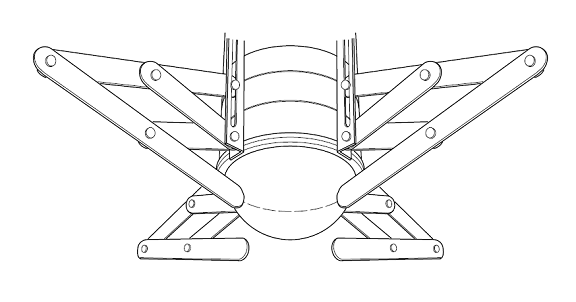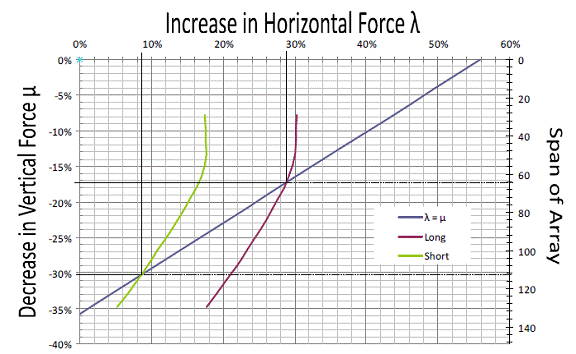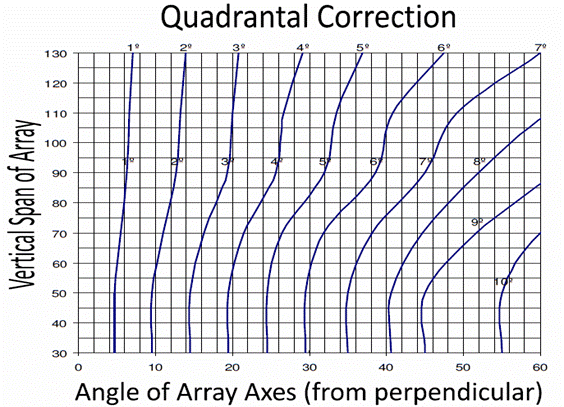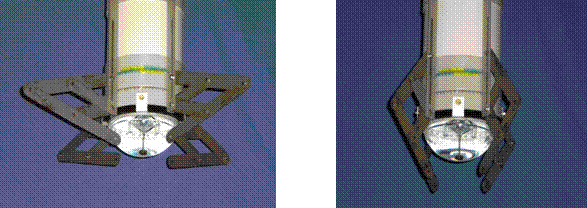The Binnacle.

The binnacle is designed to correct for horizontal and vertical induced fields affecting the mean directive force at the compass. (a,b,d,e & k rods).The quadrantal correctors comprises two pairs of soft iron scissor arrays arms, each pair mounted radially outwards from a binnacle and spaced 180º apart on a ring that can be rotated around the binnacle.

Each scissor array reduces the vertically induced field at the compass. The amount of reduction can be varied by increasing the span of the scissor array.

Each pair of scissor arrays increases the horizontal magnetic field strength along the direction of their axis and each pair alone creates quadrantal deviation. If the axes of the paired arrays are perpendicular, the horizontal field strength is increased uniformly on all headings. By adjusting the angle of the paired array’s axes the increase in horizontal field can be biased along any axis to correct for quadrantal deviation.

Using the modern post panamax vessel’s observations, the wheelhouse compass site was measured to have vertically induced fields causing a multiplier of the vertical field (µ) of 120% and horizontally induced fields causing a multiplier of the horizontal field (λ) of 77%. This is an increase of 20% in the vertical field and a decrease of 23% in the horizontal field.

To have both the vertical and horizontal fields with the same percentage of the earth’s field, the vertical field can be reduced until it is 77% of the earth’s field ((77-120)/120 = -35.8% decrease), the horizontal field can be increased until it is 120% of the earth’s field ( (120-77)/77 =  55.8% increase ) or somewhere in-between.

On a graph, the required increase in the horizontal field alone can be put on the X axis and the required decrease in the vertical field alone is put on the Y axis. A line can be drawn from the percentage of only changing the horizontal force on the X axis to only changing the vertical force on the Y axis. This line represents the corresponding values of increasing the horizontal force to decreasing the vertical force in order to deliver the same multiplier of the vertical and horizontal force to the ambient magnetic field.

The intersection of this line and the performance curve of the Quadrantal Corrector indicates the required setting of the span of the array to deliver equal multipliers to the vertical and horizontal fields.

From figure 1, it can be seen that the span of the array should be set to decrease the vertical induced field by 17½ % which will provide a corresponding increase in the horizontally induced field of 28½%. The effect of these settings is to reduce the vertical field to 99.3% of the earths field ((1-0.175) x 120 = 99.3%) and increase the horizontal field to 99.3% of the earth’s field ((1+ 0.285) x 77 = 99.3%).  As the vertically induced force from the correctors is directly proportional to the span of the array opening, the setting of the opening can be taken directly off the graph’s second Y axis. In this example, the span of the arrays should be set to 64 mm.

(If the shorter arrays were used it can be seen that the span of the arrays would be set at 113mm delivering a mean directive force of 83½ % of the earth’s field.)Figure 1

Continuing with the example from the modern post panamax, the observed quadrantal error was a minus co-efficient D of 1º. By going to the Quadrantal Correction graph (figure 2) the required bias of the array axes can be derived. In this case, the array axes each need to be brought towards fore & aft line by 3½º to bring them 7º from perpendicularity to increase the fore & aft directional force.

(If the shorter arrays were being used, they would each be set at 7º towards the fore & aft line to bring them 14º from perpendicularity.)Figure 2

Soft Iron Arrays set for correct compensation on the post panamax bulkcarrier.

Long Arrays delivering a multiplier of 99.3%                              Short Arrays delivering a multiplier of 83%